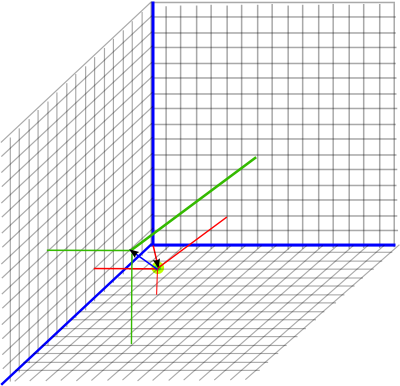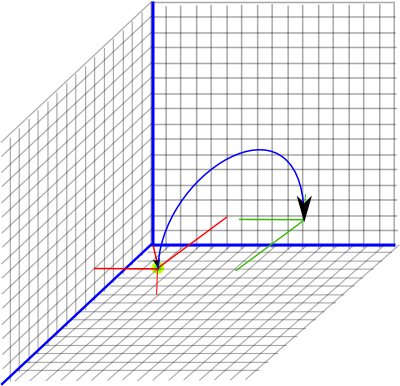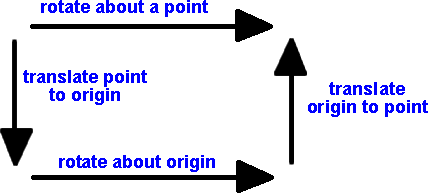# Maths - Dual Quaternions

The Dual of a Quaternion can model the movement of a solid object in 3D, which can rotate and translate without changing shape, that is distances and angles between points on the object are preserved. In mathematical terms it can be used to represent the group of spatial displacements SE(3).

We could either start with a dual and make each element of the dual a quaternion or start with a quaternion and make each element a dual. Either way the result is the same, that there are now 8 elements, the 4 quaternion elements (real, i, j and k) and their duals (ε, iε, jε and kε). This gives dual quaternions an 8x8 multiplication table as shown here:

### Table for: Dual Quaternion

 a*b b.1 b.i b.j b.k b.ε b.εi b.εj b.εk a.1 1 i j k ε εi εj εk a.i i -1 k -j εi -ε -εk εj a.j j -k -1 i εj εk -ε -εi a.k k j -i -1 εk -εj εi -ε a.e ε -εi -εj -εk 0 0 0 0 a.ei εi ε -εk εj 0 0 0 0 a.ej εj εk ε -εi 0 0 0 0 a.ek εk -εj εi e 0 0 0 0

analysing commutivity: table does not commute: for example: i*j != j*i

analysing associativity: table does not associate, for example,
(i* j)* ε=k* ε=εk is not equal to i*(j* ε)=i*εj=-εk

how these results were generated.

As the above link explains, the table was generated by a computer program using a modification of Cayley-Dickson process.

However this differs from the table usually used for dual quaternions:

 a*b b.1 b.i b.j b.k b.1ε b.iε b.jε b.kε a.1 1 i j k 1ε iε jε kε a.i i -1 k -j iε -1ε kε -jε a.j j -k -1 i jε -kε -1ε iε a.k k j -i -1 kε jε -iε -1ε a.1ε 1ε iε jε kε 0 0 0 0 a.iε iε -1ε kε -jε 0 0 0 0 a.jε jε -kε -1ε iε 0 0 0 0 a.kε kε jε -iε -1ε 0 0 0 0

The red entries have a sign change, this is caused by the following:

• The first table has ε in front of the other operators (i,j & k). This is a trivial change and it is easy to show that this change produces an algebra that is isomorphic to the original.
• In the first table ε anticommutes with the other operators (i,j & k), in the second table ε commutes with the other operators, this is a more significant difference.

The usual addition and subtraction rules apply, just add or subtract corresponding terms, the interesting properties are defined by the multiplication table.

## Representing Transforms

The advantage of this is that we can represent a combined rotation with displacement operation with one multiplication operation.

• The 1, i, j and k terms together can represent rotation (as a normalised quaternion).
• The 1ε, iε, jε and kε terms together can represent displacement (where 1ε =0 and (iε, jε, kε) is a vector equal to half the displacement)

We will do the transform using the same equation as we used for quaternion rotation:

P2=Q * P1 * Q'

where:

• P1 = vector representing the initial position of a point being transformed.
• q = the dual quaternion representing the transform
• Q' = the 3rd type of conjugate described here.
• p2 = the result which is a vector representing the final position of the point.

### Pure Displacement (no rotation)

Let

• h = a 3D vector representing half the displacement
• p1 = a 3D vector representing the initial position of a point

We will use the notation εh as a shortcut to represent the vector (x iε + y jε + z kε) and similarly for ε p1.

The transform can be done by:

Pout = Q * Pin * Q† (where Q† = r† - ε d† see this page)
(1 + ε p2) = (1 + ε h)(1 + ε p1)(1 + ε h)
= (1 + ε h + ε p1)(1 + ε h)
since e²=0 we can ignore these terms
= (1 + ε h + ε h + ε p1)
= (1 + 2 ε h + ε p1)

So this adds the displacement (2*half) to the original point which is what we want.

If the rotation is 0 degrees, the rotation part is represented by (1 + 0 i + 0 j + 0 k), in other words 1, as explained on this page.

#### Example

So if we are initially at point (x=3, y=4, z=5) this will be represented by the Dual Quaternion:

P1 = 1 + 3 iε + 4 jε + 5 kε

A pure displacement will be represented by the dual quaternion:

(1 + x/2 iε + y/2 jε + z/2 kε)

We now want to displace the initial point by (x=4, y=2, z=6) this will be represented by the Dual Quaternion:

q = (1 + 2 iε + 1 jε + 3 kε)So to combine these, to give the resulting position, we use P2 = q * P1 * q' to give:

p2 = (1 + 2 iε + 1 jε + 3 kε)*(1 + 3iε + 4jε + 5 kε)*(1 + 2 iε + 1 jε + 3 kε)

multiplying out the first two terms using the above multiplication table we get:

P2 = (1 + 5 iε + 5 jε + 8 kε)*(1 + 2 iε + 1 jε + 3 kε)
P2 = (1 + 7 iε + 6 jε + 11 kε)

### Pure Rotation (no displacement)

If the displacement is zero then iε = jε = kε = 0 and the rotation is represented by the normalised quaternion as explained on this page.#### Example

Applying a rotation of point (3,4,5) by 180° around the x axis is given by:

P2 = (0 + i)*(1 + 3iε + 4jε + 5 kε)*(0 - i)
P2 = ( i - 3ε -5jε + 4kε)*(0 - i)
P2 = 1 + 3iε - 4jε - 5 kε

### Combined Displacement and Rotation (displace then rotation)

#### Example

Starting from the previous position: (1 + 3iε + 4jε + 5 kε)

and both displace by (x=4, y=2, z=6) and applying a rotation of 180° around the x axis represented by: (0 + i)

Therefore:

Q = (0 + i) (1 + 2 iε + 1 jε + 3 kε)
Q = (i -2ε -3 jε + 1 kε)

So applying the transform gives:

P2 = (i - 2ε -3 jε + 1 kε)*(1 + 3iε + 4jε + 5 kε)*(-i + 2ε -3 jε + 1 kε)
P2 = (i - 5iε - 8jε + 5 kε)*(-i + 2ε -3 jε + 1 kε)
P2 = 1 + 7iε -6jε - 11kεIn the above we are rotating about the origin. This is sometimes what we want to do, for instance, if we are observing the world from the frame of reference of a car and we assume it to be at the origin then, when it turns, the other cars will appear to rotate round it. If we want a car to rotate about its own centre then we can just multiply the complex number part (1 and i) and ignore the e and ie part.

If we want to rotate about an arbitrary point then we first translate this point to the origin, then rotate about the origin, then translate the origin back to the point (as discussed on this page).## Combining Transforms

Q = r

Q = 1 + ε½t

Q = (1 + ε½t)*r
= r + ε½t r

### Translation then Rotation

Q = r*(1 + ε½t)
= r + ε½r t

Q = (1 + ε½c)*r*(1 - ε½c)
= (1 + ε½c)*(r - ε½r c)
= r - ε½r c + ε½c r

## Inverse of Transform

To invert the quaternion 'r' we use its conjugate 'r†', to invert the translation 't' we use '-t'. With combined transforms we must also reverse the order that they are combined (don't forget that order is important for translations and their corresponding dual quaternion).

The inverse of common transforms are:

 Q Q-1 Pure Rotation r r† Pure Translation 1 + ε½t 1 - ε½t Rotation then Translation r + ε½t r r† - ε½r† t Translation then Rotation r + ε½r t r† - ε½t r† Rotation about a point 'c' r - ε½r c + ε½c r r† + ε½r† c - ε½c r†

## Geometric Algebra Representation

The algebra, derived here as a dual whose elements are quaternions or a quaternion whose elements are duals, can also be derived as a Geometric Algebra G+3,0,1. That is a Geometric Algebra generated from a 4 dimensional vector space with 3 dimensions which square to +ve and one dimension which squares to zero. We then take the subalgebra made up of the even grade elements: scalar + 6 dimensional bivector + pseudoscalar.

The dimensions are related as follows:

 dual quaternion G+ 3,0,1 1 e i e12 j e31 k e23 kε e41 jε e42 iε e43 1ε e1234

The use of Geometric Algebra to represent this type of tranform can be analysed using conformal space as described on this page.

## Use for Physics

The reason for taking this approach is to combine linear and rotational movement into a single transform and to automatically handle the interaction between them. It would be good to be able to use this algebra to work with physics equations. For instance, imagine we have a set of forces acting on a solid body, can we determine the linear and rotational movement by using dual quaternions?

Dual Quaternions represent both the linear and rotational movement as bivectors (as we can see from the Geometric Algebra version of this algebra explained on this page). Bivectors are associated with planes, or quantities associated with them like rotations, we would expect a linier displacement to be represented by a vector. I think this issue could be a problem if we try to formulate the physics equations in terms of dual quaternions.

However linier movement can be represented as a rotation... If we rotate around a point at infinity! One way to handle this type of situation would be to use projective space as explained on this page. Is it possible to use this to do what we want? I would appreciate any help.